Yakışıklı erkek tatil için bir beldeye gidiyor burada kendisine türk Porno güzel bir seksi kadın ayarlıyor Onunla beraber otel odasına gidiyorlar Otel odasına rokettube giren kadın ilk önce erkekle sohbet ederek işi yavaş halletmeye çalışıyor sex hikayeleri Kocası fabrikatör olan sarışın Rus hatun şehirden biraz uzak olan bir türk porno kasabaya son derece lüks bir villa yaptırıp yerleşiyor Kocasını işe gönderip mobil porno istediği erkeği eve atan Rus hatun son olarak fotoğraf çekimi yapmak üzere türk porno evine gelen genç adamı bahçede azdırıyor Güzel hatun zengin bir iş adamının porno indir dostu olmayı kabul ediyor Adamın kendisine aldığı yazlık evde sikiş kalmaya başlayan hatun bir süre sonra kendi erkek arkadaşlarını bir bir çağırarak onlarla porno izle yapıyor Son olarak çağırdığı arkadaşını kapıda üzerinde beyaz gömleğin açık sikiş düğmelerinden fışkıran dik memeleri ile karşılayıp içeri girer girmez sikiş dudaklarına yapışarak sevişiyor Evin her köşesine yayılan inleme seslerinin eşliğinde yorgun düşerek orgazm oluyor film izle
All submissions of the EM system will be redirected to Online Manuscript Submission System. Authors are requested to submit articles directly to Online Manuscript Submission System of respective journal.

# Continuous Dependence of the Solution of A Stochastic Differential Equation With Nonlocal Conditions

El-Sayed AMA1*, Abd-El-Rahman RO2, El-Gendy M2

1Faculty of Science, Alexandria University, Egypt

2Faculty of Science, Damanhour University, Egypt

Corresponding Author:
El-Sayed AMA
Faculty of Science Alexandria University, Egypt
E-mail: amasyed@yahoo.com

Received date: 21/01/2016; Accepted date: 14/04/2016; Published date: 18/04/2016

Visit for more related articles at Research & Reviews: Journal of Statistics and Mathematical Sciences

## Abstract

In this paper we are concerned with a nonlocal problem of a stochastic differential equation that contains a Brownian motion. The solution contains both of mean square Riemann and mean square Riemann-Steltjes integrals, so we study an existence theorem for unique mean square continuous solution and its continuous dependence of the random data X0 and the (non-random data) coefficients of the nonlocal condition ak. Also, a stochastic differential equation with the integral condition will be considered.

#### Keywords

Integral condition, Brownian motion, Unique mean Square solution, Continuous dependence, Random data, Non- Random data, Integral condition

#### Introduction

Many authors in the last decades studied nonlocal problems of ordinary differential equations, the reader is referred to [1-7], and references therein. Also the theory of stochastic differential equations, random fixed point theory, existence of solutions of stochastic differential equations by using successive approximation method and properties of these solutions have been extensively studied by several authors, especially those contain the Brownian motion as a formal derivative of the Gausian white noise, the Brownian motion W (t), t ∈ R, is defined as a stochastic process such that

W (0) = 0; E(W (t)) = 0, E(W (t))2 = t

and [W (t1) W (t2)] is a Gaussian random variable for all t1, t2 ∈ R. The reader is referred to [8,9] and [10-16] and references therein.

Here we are concerned with the stochastic differential equation

dX(t) = f (t, X(t))dt + g(t)dW (t), t ∈ (0, T ] (1)

with the nonlocal random initial condition(2)

where X0 is a second order random variable independent of the Brownian motion W (t) and ak are positive real integers. The existence of a unique mean square solution will be studied. The continuous dependence on the random data X0 and the nonrandom data ak will be established. The problem (1) with the integral condition will be considered.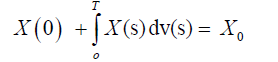(3)

#### Integral Representation

Let C = C(I, L2( Ω)) be the class of all mean square continuous second order stochastic process with the normThroughout the paper we assume that the following assumptions hold

(H1) The functionis mean square continuous.

(H2) There exists an integrable function k : [0, T ] → R+ , wheresuch that the function f satisfies the mean square Lipschitz condition(H3) There exists a positive real number m1 such thatNow we have the following lemmas.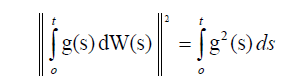#### Proof.This completes the proof.

Lemma 2.2: The solution of the problem (1) and (2) can be expressed by the integral equation(4)

whereProof. Integrating equation (1), we obtainand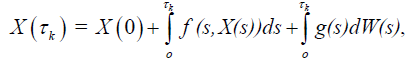thenandthenHenceNow define the mappingThen we can prove the following lemma.

Lemma 2.3 F : C → C.

Proof.Let 1 2 X ∈C, t , t ∈ [0, T] such that 2 1 t -t <δ , then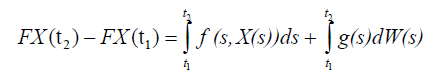From assumption (ii) we have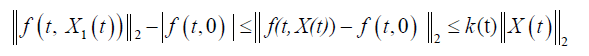then we haveSo,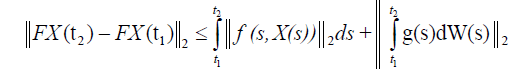using assumptions and lemma 2.1, we getwhich proves that F : C → C.

#### Existence and Uniqueness

For the existence of a unique continuous solution X ∈ C of the problem (1)-(2), we have the following theorem.

Theorem 3.1 Let the assumptions (H1)−(H3) be satisfied. If 2m < 1, then the problem(1)-(2) has a unique solution X ∈ C.

Proof. Let X and X* ∈ C, thenHence

If 2m < 1 , then F is contraction and there exists a unique solution X ∈ C of the nonlocal stochastic problem (1)-(2), . This solution is given by (4)#### Continuous Dependence

Consider the stochastic differential equation (1) with the nonlocal conditionDefinition 4.1 The solution X ∈ C of the nonlocal problem (1)-(2) is continuously dependent (on the data X0) ifsuch thatimplies thatHere, we study the continuous dependence (on the random data X0) of the solution of the stochastic differential equation (1) and (2).

Theorem 4.2 Let the assumptions (H1) − (H3) be satisfied. Then the solution of the nonlocal problem (1)-(2) is continuously dependent on the random data X0.

Proof. Letbe the solution of the nonlocal problem (1)-(2) andbe the solution of the nonlocal problem (1) and (6). ThenUsing our assumptions, we getthenThis completes the proof.

Now consider the stochastic differential equation (1) with the nonlocal conditionDefinition 4.2 The solution X ∈ C of the nonlocal problem (1)-(2) is continuously dependent (on the coefficient ak of the nonlocal condition) ifsuch thatimplies that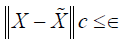Here, we study the continuous dependence (on the random data X0) of the solution of the stochastic differential equation (1) and (2).

Theorem 4.3 Let the assumptions (H1) − (H3) be satisfied. Then the solution of the nonlocal problem (1)-(2) is continuously dependent on the coefficient ak of the nonlocal condition.

Proof. Letbe the solution of the nonlocal problem (1)-(2) andbe the solution of the nonlocal problem (1) and (7). Then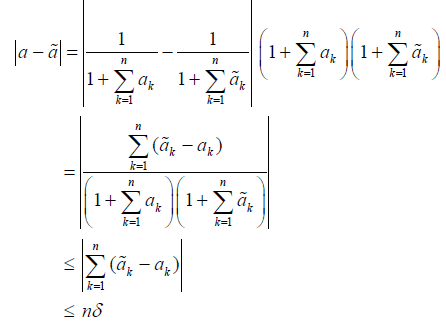andand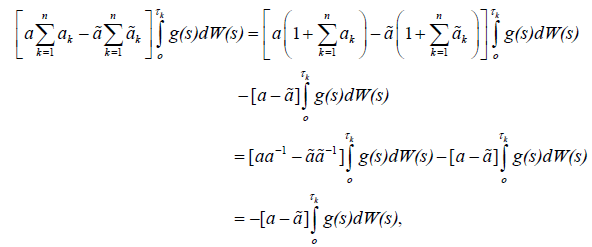Then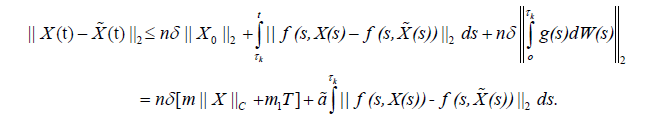Using our assumptions we getthen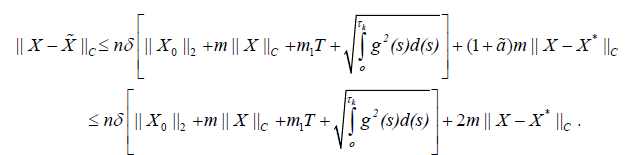HenceThis completes the proof.

#### Non Local Integral Condition

Let ak = v(tk) − v(tk −1), τk ∈ (tk−1, tk), where (0 < t1 < t2 < t3 < …< T).

Then, the nonlocal condition (2) will be in the formFrom the mean square continuity of the solution of the nonlocal problem (1)-(2), we obtain fromthat is, the nonlocal conditions (2) is transformed to the mean square Riemann-Steltjes integral conditionNow, we have the following theorem.

Theorem 5.4 Let the assumptions (H1)-(H3) be satisfied, then the stochastic differential equation (1) with the nonlocal integral condition (3) has a unique mean square continuous solution represented in the formProof. Taking the limit of equation (4) we get the proof.